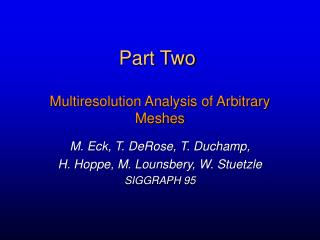DownloadDownload PresentationPart Two

# Part Two

Download Presentation## Part Two

- - - - - - - - - - - - - - - - - - - - - - - - - - - E N D - - - - - - - - - - - - - - - - - - - - - - - - - - -
##### Presentation Transcript

1. Part Two Multiresolution Analysis of Arbitrary Meshes M. Eck, T. DeRose, T. Duchamp, H. Hoppe, M. Lounsbery, W. Stuetzle SIGGRAPH 95

2. Outline • Partition using Voronoi diagram and Delaunay triangulation • Parameterization using Harmonic Maps Original mesh Base complex

3. Applications • Remeshing – subdivision connectivity • Multiresolution analysis and editing • Level-of-detail

4. Harmonic Maps • Defined for topological disks • Minimize distortion in some sense • Do not guarantee an embedding (triangles flips may occur) • Require boundary conditions

5. Harmonic Maps - definition Input: mesh G with disk topology: N vertices {p1, …, pn , …, pN} where p1, …, pnare boundary vertices Goal: Compute an embedding h of G h(i) = qi = (ui , vi) i = 1, …, N

6. Harmonic Maps - definition • The boundary vertices are mapped to a convex polygon in the parameter domain • Treat each mesh edge as a spring, find mappings for inner vertices s.t. the energy of the spring system is minimal

7. Boundary conditions - corner vertices

8. Mapping inner vertices

9. Harmonic mapping • Strives to preserve angles (conformal) • We treat the mesh as a system of springs. • Define spring energy: where vi are the flat positions, and the boundary vertices v1, v2, …, vn are constrained.

10. Energy minimization – least squares • We want to find such flat positions that the energy is as small as possible. • Solve the linear least squares problem! Eharmis function of 2(N–n) variables

11. Energy minimization – least squares • To find minimum: Eharm= 0 • Again, x1,…., xn and y1, …, yn are constrained.

12. Energy minimization – least squares • To find minimum: Eharm= 0 • Again, x1,…., xn and y1, …, yn are constrained.

13. The spring constants ki,j • The weights ki,j are chosen to minimize angles distortion: • Look at the edge (i, j) in the 3D mesh • Set the weight ki,j = cot  + cot i   3D j

14. Partition • Compute Voronoi diagram on the mesh • Compute induced Delaunay triangulation • Construct the base mesh

15. Voronoi Diagram • Given s faces (= sites) on the surface, expand patches (= Voronoi cells) around each one, using s-source Dijkstra algorithm • How to choose the sites? • Each Voronoi cell must be a topologic disk • Pair of cells share at most one cut • No more than three cells share a vertex

16. Voronoi Diagram

17. Voronoi Diagram Each cell is a topological disk

18. Voronoi Diagram Two cells share at most one boundary

19. Voronoi Diagram Two cells share at most one boundary

20. Voronoi Diagram At most three cells meet at a vertex

21. Choosing the sites – greedy approach Let S be the set of sites. Initiate S with a random triangle on the mesh. • Grow the cells around the sites of S until one of the conditions is violated. • Add the violating face to S and repeat the process.

22. Constructing Delaunay triangulation • On the original mesh: connect by a path each pair of sites whose cells are adjacent • To construct the base mesh: connect each pair of adjacent sites by a straight line.

23. Constructing Delaunay triangulation

24. Constructing Delaunay triangulation

25. Base mesh

26. Algorithm summary • Partition the mesh (Delaunay triangulation) • Construct the base mesh • Parameterize each patch over the corresponding face of the base mesh. The mappings of adjacent patches agree on the boundary. Continuous parameterization over the base mesh!

27. Results

28. 34 min. , 70,000 faces 4,600 faces , e < 1.2 % 162 faces 2,000 faces , e < 2.0 %

29. 40 min. , 100,000 faces 4,700 faces , e < 1.5 % 229 faces 2,000 faces , e < 2.0 %

30. 346 min. , 166,000 faces 16,451 faces , e < 2.6 % 132 faces

31. Texture mapping

32. Convex parameterization

33. Convex weights for inner vertices • If the weights are convex, the solution is always valid (no self-intersections) [Floater 97] • The cotangent weight in Harmonic Mapping can be negative  sometimes there are triangle flips

34. End of Part Two